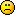Hi

I build strategy with RSI and I want to sell at above 80 and buy at below 20
I found out that it is doing also long on above 80 and short below 20.
This is what written in Strategy Overview for example
Opening (Entry Signal)
Open a new long position at the beginning of the bar when the following logic condition is satisfied:
•    the RSI* (Simple, Close, 7) is higher than the Level 80.
Open a new short position at the beginning of the bar when the following logic condition is satisfied:
•    the RSI* (Simple, Close, 7) is lower than the Level 20.

It should be the opposite!!!!Short above 80 long below 20

Is it my mistake?
I’ll appreciate any help
BTW – GREAT GREAT TOOL !!!!
Nir

Hello Nir,

There is no problem,
You have to set long position's logic in the strategy slots.

If you want to buy when RSI < 20 and to sell when RSI > 80, you have to set the rule for the long entry only. In this case the rule should be: Enter long when RSI is lower than 20. This also means that the short entry is when RSI is higher than 80.

[Opening Point of the Position]
Bar Opening
Enter the market at the beginning of the bar
Base price  -  Open

[Opening Logic Condition]
RSI
The RSI is lower than the Level line  <---------------
Smoothing method  -  Simple
Base price  -  Close
Smoothing period  -  14
Level  -  20         <---------------
Use previous bar value  -  Yes

In the "Strategy Overview":

Opening (Entry Signal)

* Open a new long position at the beginning of the bar when the following logic condition is satisfied:

the RSI* (Simple, Close, 14) is lower than the Level 20.

* Open a new short position at the beginning of the bar when the following logic condition is satisfied:

the RSI* (Simple, Close, 14) is higher than the Level 80.